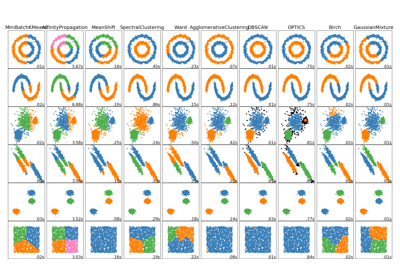# sklearn.cluster.SpectralClustering¶

class sklearn.cluster.SpectralClustering(n_clusters=8, eigen_solver=None, random_state=None, n_init=10, gamma=1.0, affinity=’rbf’, n_neighbors=10, eigen_tol=0.0, assign_labels=’kmeans’, degree=3, coef0=1, kernel_params=None, n_jobs=None)[source]

Apply clustering to a projection of the normalized Laplacian.

In practice Spectral Clustering is very useful when the structure of the individual clusters is highly non-convex or more generally when a measure of the center and spread of the cluster is not a suitable description of the complete cluster. For instance when clusters are nested circles on the 2D plane.

If affinity is the adjacency matrix of a graph, this method can be used to find normalized graph cuts.

When calling fit, an affinity matrix is constructed using either kernel function such the Gaussian (aka RBF) kernel of the euclidean distanced d(X, X):

np.exp(-gamma * d(X,X) ** 2)


or a k-nearest neighbors connectivity matrix.

Alternatively, using precomputed, a user-provided affinity matrix can be used.

Read more in the User Guide.

Parameters: n_clusters : integer, optional The dimension of the projection subspace. eigen_solver : {None, ‘arpack’, ‘lobpcg’, or ‘amg’} The eigenvalue decomposition strategy to use. AMG requires pyamg to be installed. It can be faster on very large, sparse problems, but may also lead to instabilities. random_state : int, RandomState instance or None (default) A pseudo random number generator used for the initialization of the lobpcg eigen vectors decomposition when eigen_solver='amg' and by the K-Means initialization. Use an int to make the randomness deterministic. See Glossary. n_init : int, optional, default: 10 Number of time the k-means algorithm will be run with different centroid seeds. The final results will be the best output of n_init consecutive runs in terms of inertia. gamma : float, default=1.0 Kernel coefficient for rbf, poly, sigmoid, laplacian and chi2 kernels. Ignored for affinity='nearest_neighbors'. affinity : string, array-like or callable, default ‘rbf’ If a string, this may be one of ‘nearest_neighbors’, ‘precomputed’, ‘rbf’ or one of the kernels supported by sklearn.metrics.pairwise_kernels. Only kernels that produce similarity scores (non-negative values that increase with similarity) should be used. This property is not checked by the clustering algorithm. n_neighbors : integer Number of neighbors to use when constructing the affinity matrix using the nearest neighbors method. Ignored for affinity='rbf'. eigen_tol : float, optional, default: 0.0 Stopping criterion for eigendecomposition of the Laplacian matrix when eigen_solver='arpack'. assign_labels : {‘kmeans’, ‘discretize’}, default: ‘kmeans’ The strategy to use to assign labels in the embedding space. There are two ways to assign labels after the laplacian embedding. k-means can be applied and is a popular choice. But it can also be sensitive to initialization. Discretization is another approach which is less sensitive to random initialization. degree : float, default=3 Degree of the polynomial kernel. Ignored by other kernels. coef0 : float, default=1 Zero coefficient for polynomial and sigmoid kernels. Ignored by other kernels. kernel_params : dictionary of string to any, optional Parameters (keyword arguments) and values for kernel passed as callable object. Ignored by other kernels. n_jobs : int or None, optional (default=None) The number of parallel jobs to run. None means 1 unless in a joblib.parallel_backend context. -1 means using all processors. See Glossary for more details. affinity_matrix_ : array-like, shape (n_samples, n_samples) Affinity matrix used for clustering. Available only if after calling fit. labels_ : Labels of each point

Notes

If you have an affinity matrix, such as a distance matrix, for which 0 means identical elements, and high values means very dissimilar elements, it can be transformed in a similarity matrix that is well suited for the algorithm by applying the Gaussian (RBF, heat) kernel:

np.exp(- dist_matrix ** 2 / (2. * delta ** 2))


Where delta is a free parameter representing the width of the Gaussian kernel.

Another alternative is to take a symmetric version of the k nearest neighbors connectivity matrix of the points.

If the pyamg package is installed, it is used: this greatly speeds up computation.

References

Examples

>>> from sklearn.cluster import SpectralClustering
>>> import numpy as np
>>> X = np.array([[1, 1], [2, 1], [1, 0],
...               [4, 7], [3, 5], [3, 6]])
>>> clustering = SpectralClustering(n_clusters=2,
...         assign_labels="discretize",
...         random_state=0).fit(X)
>>> clustering.labels_
array([1, 1, 1, 0, 0, 0])
>>> clustering
SpectralClustering(affinity='rbf', assign_labels='discretize', coef0=1,
degree=3, eigen_solver=None, eigen_tol=0.0, gamma=1.0,
kernel_params=None, n_clusters=2, n_init=10, n_jobs=None,
n_neighbors=10, random_state=0)


Methods

 fit(self, X[, y]) Creates an affinity matrix for X using the selected affinity, then applies spectral clustering to this affinity matrix. fit_predict(self, X[, y]) Performs clustering on X and returns cluster labels. get_params(self[, deep]) Get parameters for this estimator. set_params(self, \*\*params) Set the parameters of this estimator.
__init__(self, n_clusters=8, eigen_solver=None, random_state=None, n_init=10, gamma=1.0, affinity=’rbf’, n_neighbors=10, eigen_tol=0.0, assign_labels=’kmeans’, degree=3, coef0=1, kernel_params=None, n_jobs=None)[source]
fit(self, X, y=None)[source]

Creates an affinity matrix for X using the selected affinity, then applies spectral clustering to this affinity matrix.

Parameters: X : array-like or sparse matrix, shape (n_samples, n_features) OR, if affinity==precomputed, a precomputed affinity matrix of shape (n_samples, n_samples) y : Ignored
fit_predict(self, X, y=None)[source]

Performs clustering on X and returns cluster labels.

Parameters: X : ndarray, shape (n_samples, n_features) Input data. y : Ignored not used, present for API consistency by convention. labels : ndarray, shape (n_samples,) cluster labels
get_params(self, deep=True)[source]

Get parameters for this estimator.

Parameters: deep : boolean, optional If True, will return the parameters for this estimator and contained subobjects that are estimators. params : mapping of string to any Parameter names mapped to their values.
set_params(self, **params)[source]

Set the parameters of this estimator.

The method works on simple estimators as well as on nested objects (such as pipelines). The latter have parameters of the form <component>__<parameter> so that it’s possible to update each component of a nested object.

Returns: self

## Examples using sklearn.cluster.SpectralClustering¶Comparing different clustering algorithms on toy datasets